Get inspired by the success stories of our students in IIT JAM MS, ISI  MStat, CMI MSc DS.  Learn More

# Discover the Covariance | ISI MStat 2016 Problem 6This problem from ISI MStat 2016 is an application of the ideas of indicator and independent variables and covariance of two summative random variables.

## Problem- Covariance Problem

Let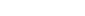~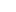be i.i.d. random variables from a continuous distribution whose density is symmetric around 0. Suppose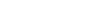. Define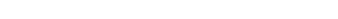.
Calculate the covariance between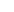and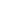.

This problem is from ISI MStat 2016 (Problem #6)

### Prerequisites

1. X has Symmetric Distribution around 0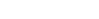.
2.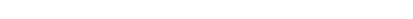, whereis a random variable.
3.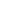and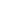are independent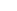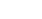and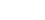are independent.
4.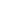andare independent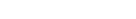.

## Solution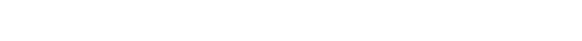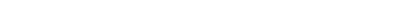.

Let's calculate the covariance ofand.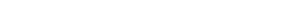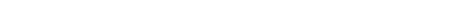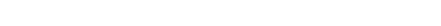.

This problem from ISI MStat 2016 is an application of the ideas of indicator and independent variables and covariance of two summative random variables.

## Problem- Covariance Problem

Let~be i.i.d. random variables from a continuous distribution whose density is symmetric around 0. Suppose. Define.
Calculate the covariance betweenand.

This problem is from ISI MStat 2016 (Problem #6)

### Prerequisites

1. X has Symmetric Distribution around 0.
2., whereis a random variable.
3.andare independentandare independent.
4.andare independent.

## Solution.

Let's calculate the covariance ofand..

This site uses Akismet to reduce spam. Learn how your comment data is processed.

### Knowledge Partner